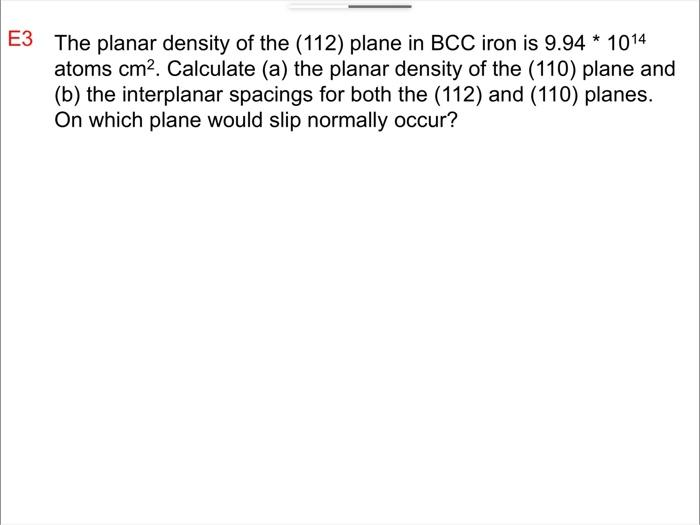# Question 3 The planar density of the (112) plane in BCC iron is $$9.94 * 10^{14}$$ atoms $$\mathrm{cm}^{2}$$. Calculate (a) the planar density of the (110) plane and (b) the interplanar spacings for both the (112) and (110) planes. On which plane would slip normally occur?KVEJ2P The Asker · Electrical EngineeringTranscribed Image Text: 3 The planar density of the (112) plane in BCC iron is $$9.94 * 10^{14}$$ atoms $$\mathrm{cm}^{2}$$. Calculate (a) the planar density of the (110) plane and (b) the interplanar spacings for both the (112) and (110) planes. On which plane would slip normally occur?
More
Transcribed Image Text: 3 The planar density of the (112) plane in BCC iron is $$9.94 * 10^{14}$$ atoms $$\mathrm{cm}^{2}$$. Calculate (a) the planar density of the (110) plane and (b) the interplanar spacings for both the (112) and (110) planes. On which plane would slip normally occur?